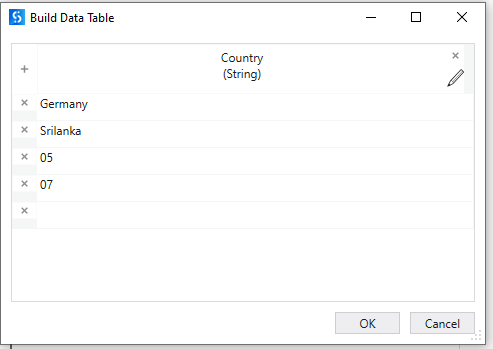# Get numeric value from row data in the excel then if numeric present in that row then we need to send the exception

Hi
I want to extract the numeric value from the row column, if it is numeric in the column then it should throw a exception.
Help

Hi @Vardhan1 ,

Could you let us know are you trying to check the value in a specific row column or Do you want to check all or any of the row values contains a Number?

row name: Country
column values are germany, Srilanka, 05,07,09
So if i have a numeric values(05,07) in the country then i need to throw a mail

Assuming the below is the Representation of your Excel sheet :You could check by performing the below :

1. Read the Excel sheet using `Workbook Read Range` activity. Assign the Output a Datatable variable, say `DT`.

2. Next, we can use the below Expression to check if the column contains a numeric value.

``````DT.AsEnumerable.Any(Function(x)x("Country").ToString.IsNumeric)
``````

You can use the `If` Condition activity and place the Expression in it, in `Then` Part of the If you can place the Send Mail activity.

“in_TransactionItem.SpecificContent(item) is”
Item is column name in the input file, i have assigned the variable for the column name is item
So here i want write expression as numeric. if numeric value present in that column

then you can write condition as

``````in_TransactionItem.SpecificContent(item).ToString.IsNumeric
``````

Regards